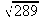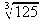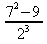MS 095 PreAlgebra Spring 2005 Quiz 2 · Name:

1. _______ Calculate:2. _______ Calculate or determine:3. _______ Determine: 19 ÷ 0

4. _______ If I run at a pace of 6.5 minutes per kilometer with a heart rate of 150 beats per minute, how many beats of my heart occur over a distance of one kilometer?

5. _______ Determine:I regularly run a 6.441 kilometer loop from Dolihner down past Palm Terrace, a left at Angies, another left into Pohn Rakied, down the hill into Kepinle, across to the Catholic mission, through the mission and around the back of the ball field to main, up main, past the Botanic Garden and on up to PICS, then on around past SDA and the Bahai temple, making a left back down into Dolihner. The following are some of my times in minutes for this loop:

44 minutes
43 minutes
47 minutes
42 minutes
46 minutes
44 minutes
49 minutes
41 minutes

_______ Determine the minimum.

1. _______ Determine the maximum.

2. _______ Determine the range.

3. _______ Determine the mode.

4. _______ Calculate the median.

5. _______ Determine the count of the data.

6. _______ Calculate the sum of the data.

7. _______ Calculate the mean (average).

8. _______ Toughie: Divide the mean time above by 6.441 kilometers to determine my average pace in minutes per kilometer for this loop.